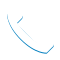Call :+91 8055223360 | 8103400400# MATLAB

MATLAB  is a high-level language and interactive environment for numerical computation, visualization, and programming. Using MATLAB, you can analyze data, develop algorithms, and create models and applications. The language, tools, and built-in math functions enable you to explore multiple approaches and reach a solution faster than with spreadsheets or traditional programming languages, such as C/C++ or Java™. You can use MATLAB for a range of applications, including signal processing and communications, image and video processing, control systems, test and measurement, computational

Duration of Training : 40 hours

Purpose

To Pipeline Engineering, Plant Design And Analysis.

Job Zones

Caesar can be used to design any type of plant—from petrochemical plants, offshore platforms, chemical and pharmaceutical plants, consumer products (food, beverages,  cosmetics, soap,  paper, and so forth), to power plants, waste water treatment plants, and cogeneration facilities.

Qualifications

ITI, ITC, VHSS, Graduates, Diploma, Engineering Students.

Production,Mechanical, Chemical

Content

Sketch, 3d Design, Analysis, Isometrics, Analysis,Programming.

Study material

E Books Pdf & References

Matlab Training Syllabus

a. History and Introduction

b. Applications in different domains

c. Overall Architecture and System Requirements

2. User Interface and Basics.

a. MATLAB UI

b. Basic Commands and usage

e. Stateflow and other toolbox.

3. MATLAB Variables

4. MATLAB and Sequences

5. User Defined Functions and Operators

6. Basic Plotting with MATLAB

7. Vectors and Matrices using MATLAB

8. Debugging with MATLAB

9. Block diagrams of Signal and Systems

10. Simulink, Stateflow and Other Toolbox

11. Problem Solving with Applications using MATLAB/ Simulink/ StateFlow:

i. Engineering Domain (Automotive + Control Systems + Electronics + Electrical Engg +

Computer Science Engineering)

ii. Financial Domain

iii. Data Analysis and applications

12. Introduction to Embedded Systems and MATLAB/Simulink.Call Us